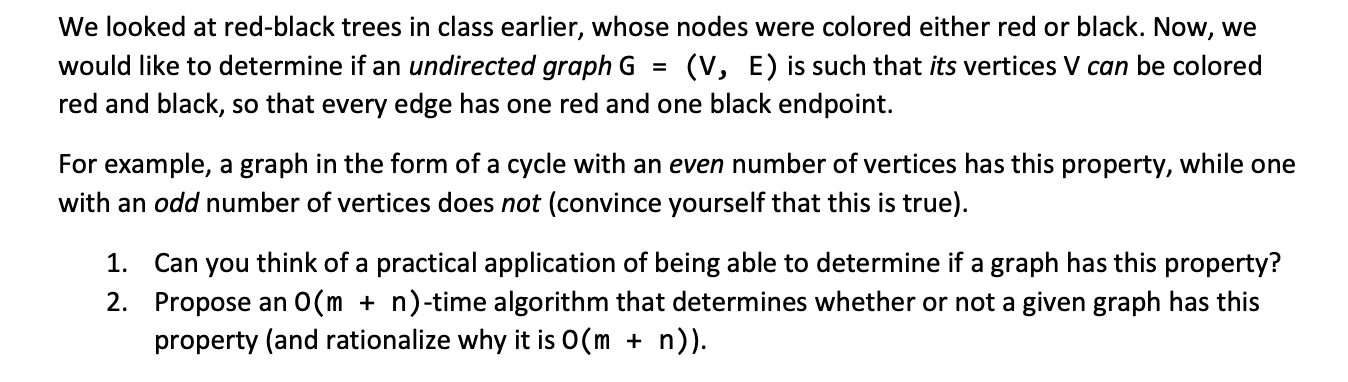# Question We looked at red-black trees in class earlier, whose nodes were colored either red or black. Now, we would like to determine if an undirected graph $$G=(V, E)$$ is such that its vertices $$V$$ can be colored red and black, so that every edge has one red and one black endpoint. For example, a graph in the form of a cycle with an even number of vertices We looked at red-black trees in class earlier, whose nodes were colored either red or black. Now, we would like to determine if an undirected graph $$G=(V, E)$$ is such that its vertices $$V$$ can be colored red and black, so that every edge has one red and one black endpoint. For example, a graph in the form of a cycle with an even number of vertices has this property, while one with an odd number of vertices does not (convince yourself that this is true). 1. Can you think of a practical application of being able to determine if a graph has this property? 2. Propose an $$O(m+n)$$-time algorithm that determines whether or not a given graph has this property (and rationalize why it is $$O(m+n)$$ ).E93BY7 The Asker · Computer ScienceTranscribed Image Text: We looked at red-black trees in class earlier, whose nodes were colored either red or black. Now, we would like to determine if an undirected graph $$G=(V, E)$$ is such that its vertices $$V$$ can be colored red and black, so that every edge has one red and one black endpoint. For example, a graph in the form of a cycle with an even number of vertices has this property, while one with an odd number of vertices does not (convince yourself that this is true). 1. Can you think of a practical application of being able to determine if a graph has this property? 2. Propose an $$O(m+n)$$-time algorithm that determines whether or not a given graph has this property (and rationalize why it is $$O(m+n)$$ ).
More
Transcribed Image Text: We looked at red-black trees in class earlier, whose nodes were colored either red or black. Now, we would like to determine if an undirected graph $$G=(V, E)$$ is such that its vertices $$V$$ can be colored red and black, so that every edge has one red and one black endpoint. For example, a graph in the form of a cycle with an even number of vertices has this property, while one with an odd number of vertices does not (convince yourself that this is true). 1. Can you think of a practical application of being able to determine if a graph has this property? 2. Propose an $$O(m+n)$$-time algorithm that determines whether or not a given graph has this property (and rationalize why it is $$O(m+n)$$ ).